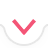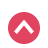# Limits

Quiz History

Select Topics
Basic

Moderate

Start Quiz
Quiz Assignment

00:00:00Summary
Previous Question

Show Hints

3 / 3
Sorry, your browser does not support this application
x^2
x^3
x^{\msquare}
\frac{\msquare}{\msquare}
\sqrt{\square}
\nthroot[\msquare]{\square}
\log_{\msquare}
\ln
\pi
\theta
\infty
\mathrm{diverges}
Let's Try Again :(
Try to simplify further
Thank you!

## Related Tutorials and Articles

Limits: The Basics
Infinite Limits and Divergence
Limits at Infinity
Limits: Factoring
Limits: Square Roots
Limits: Rational Functions
Limits: L'Hopital's Rule
Limits: The Chain Rule
Limits: The Squeeze Theorem
Cheat Sheets
Start Quiz 00:00:00
Previous Question
1 / 10
x^2
x^3
x^{\msquare}
\frac{\msquare}{\msquare}
\sqrt{\square}
\nthroot[\msquare]{\square}
\log_{\msquare}
\ln
\pi
\theta
\infty
\mathrm{diverges}
Finish Quiz

Symbolab

# Summary

Submit Assignment Start Over Back

# Summary

Start Again Back
Prev Student Next Student

in 01:12:13 hoursBack
Back

## Steps

Sorry, your browser does not support this application
Edit Quiz
Quiz Saved!# Crowns

You haven't collected any crowns yet

# Quizzes